# How To Use

INSTRUCTIONS AND STRATEGIES USING “HAYMIK HELPERS”
H10, H20, H30, H100, MULTIPLIERS 2X and 6X and FRACTIONS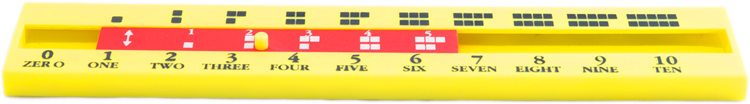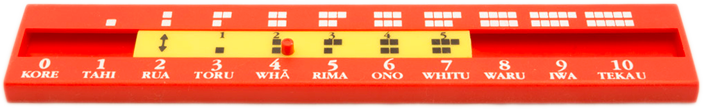HAYMIK H10 FRAMES HELPER (English or bilingual version)
Moving, the arrow on the slide over a number on the bottom board, children can visualise what quantity each number stands for, by counting the dots as they move the slide up to five on the top board.
Later they can move on by counting on the bottom board units up to ten using the dots on the top board
Learning that two is one more than one, two more than zero, one less than three, three is one more than two, two more than one, and three more than zero, these basic facts are easy to learn by manipulative and visualisation processes learning near numbers, after and before’s   (The near numbers are numbers within one or two of any given number: for example 3 & 4 to 5 & 6 ….. Simple addition can be learnt by counting on/up, as above 2 + 1 = 3 with the arrow on the yellow slide pointing to two the child counts two, three the answer above or below number “1” on the yellow slide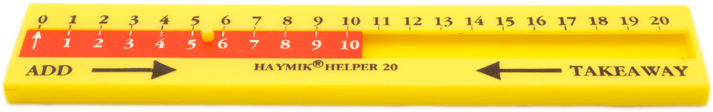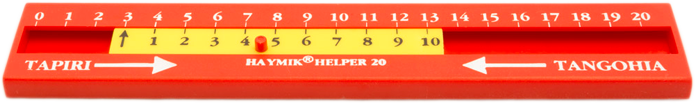HAYMIK H20 HELPER (English or bilingual version)
The “Haymik Helper” works by moving the “Arrow” on the Slide under a number on the Top Board, the answer to the sum of any number above the arrow will be found above the numbers on the slide so aligned to it on the top board.
As in the above "HAYMIK HELPER" with the“arrow on the yellow slide, pointing to number 3 on the top board and the number 2 on the yellow slide is aligned under the number 5 the answer for the sum of 3 + 2 as shown on the top board. The same principle, in reverse, works for subtraction.
Moving the arrow on the yellow slide under 2 on the top board, number 3 on the yellow slide is aligned with number 5 on the top board the sum (answer) to 2 + 3 = 5, showing us the COMMUTATIVE PROPERTY OF ADDITION which means that the order of two numbers does not affect the sum. For example 3 + 2 = 2 + 3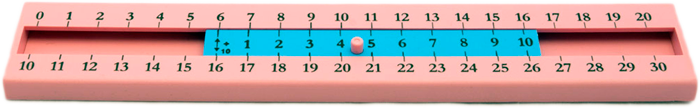INSTRUCTION for HAYMIK “30”
Extending the Number System and its Notation (Tens Notation)
Your students can now advance to the next level, of adding or subtracting two digit or more numbers and become familiar with the tens notation and sequence of numbers. The Haymik H30 works by aligning the double headed arrow on the slide with a number on the top or bottom board.  To add 16 and 7 move the arrow on the blue slide under 6 on the top board, reading off the slide 7 becomes aligned with 13 on the top board , to add the ten’s the sum 23 is aligned under 7 on the bottom board. 16+7=
= (10 +6) +7  Partitioning
= 10 + (6 + 7) Regrouping
= 22               Sum
Three-number addition depends on the regrouping of numbers to make addition easier, the fact that such regrouping is possible is known as the associative law for addition.  This states that for any numbers
A, B and C, (A + B) + C = A + (B + C).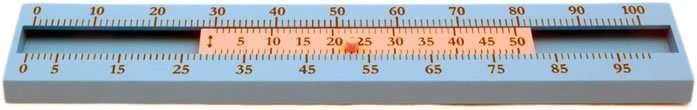HAYMIK H100 HELPER
The Haymik H100 is a versatile tool to help children with numbers up 100, skip counting by five’s and ten’s, to learn clever techniques and strategies to solve mathematical problems such as: partitioning, (using ten’s and five’s) reversibility, rounding and compensation (using neat numbers), double and near doubles, number properties (Associative, Commutative and Distributive).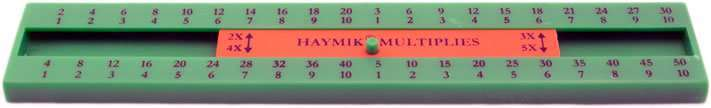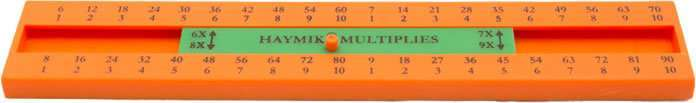Haymik Multipliers (2) 2X-5X and 6X-9X
To use skip-counting as one strategy to learn multiplication, with the 2 X arrow on the orange slide aligned under 1 move the  arrow and count  up in two’s i.e.. 2, 4, 6, 8, 10, 12, 14, 16, 18, 20, using the number sequence on the top row. You may ask how does this help,  let me explain, the number 2 on the orange slide is the first factor, the X implies times (multiply) the 7 number ( second factor) above the arrow on the green board  to obtain the product 14 ( answer ) on the top row, suggests 2 X 7 =14. How you ask, well to put it simply, multiplication is repeated addition, the first factor 2 tells us the number of groups; the second factor 7 tells us how many objects should be in each group. The product 14 is the total number of objects, 7 + 7 = 14 this example would be read as; “two groups of seven is equal to fourteen”, using multiplication, we write 2 × 7 = 14(read “two times seven is equal to fourteen)
Skip counting helps as prelude to learning their times table: Using the arrows on the slide to point to the top or bottom row of numbers, say for example on the 5 X rule, pointing to the bottom board using the top row of numbers moving the slide, count up in fives, 5, 10, 15, and 20 and so on, children can learn to count by two’s, three’s, four’s, five’s six’s, etc. on the corresponding board. Using the knob on the slide, align the double headed arrow over or under a number on the appropriate board you wish to multiply by the selected number on the middle slide.  With the arrow aligned with 5 reading off the middle slide 3X shows 3X5=15 above 5 on the top board and 5X5=25 on the bottom board and the inverse 5 into 25 = 5 on the bottom row.  The times table works the same on the other slide rules. Division is the inverse operation of multiplication, i.e.. 15 ÷ 3 = 5 in the above slide rule.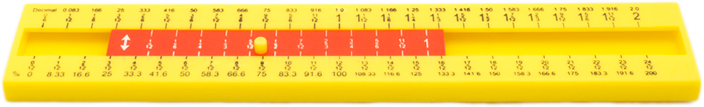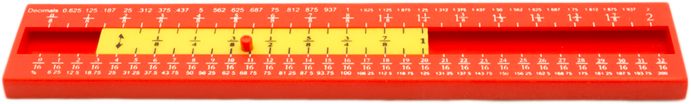Haymik Fraction Helpers (2)  One Twelfth and One Sixteenth
The fractions, decimal or percentage equivalents are found aligned under or over the double headed arrow on slide when moved on the appropriate slide rule.  To add a fraction align the arrow on the slide over or under the first fraction to be added to the second fraction on the slide the product (answer) is aligned to the second fraction on the side, above or below that fraction on the top or bottom board.
To add ? and ¼ you need to add their equivalents, to find the fraction equivalent for ¼ it will be aligned above or below ¼ on the slide i.e.., make sure the arrow is on zero. Now you add ? + = ?   to check this as above to add ? and ¼ the arrow on is aligned to ? on the top board and the second fraction ¼ on the slide in aligned to the product (answer) ?+¼ = ? on the top board.

# HAYDEN and MIKYLA

TALKING ABOUT STRATEGIES AN CLEVER TECHNIQUES LEARNT
Mikyla says to Hayden

“To add two numbers say 7 and 8, I’ll move the arrow under the first number 7 on the top board, the sum or answer is above the second number 8 on the slide. Which is 15”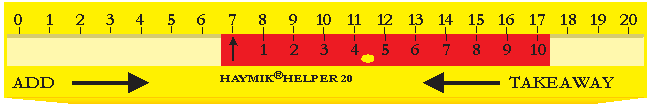Hayden says: “Mikyla I’ll use doubles or near doubles strategy for that, using the two times table and making use of number properties, look I’ll show you. See, with the arrow under 7 and 8 next to it which means 8 is one more and seven, see on the slide, number 1, is under number 8 shows 7 + 1 = 8 or 8-1 = 7 by the way it is  the commutative law for addition I’ll tell you more about this later, but back to the doubles with the difference of one between 7 and 8 I can go 2 X 7 = 14 +1 gives me 15 the sum of 7and 8  You could also go two times 8 = 16 - 1 =15 as we have already found that 8-1=7 Now back to this commutative law of addition which states that the order of the two addends makes no difference to the sum, look move the arrow under 8 now we find 15 above 7 on the slide therefore 7 + 8 = 8 + 7 showing 15 the sum in both cases.”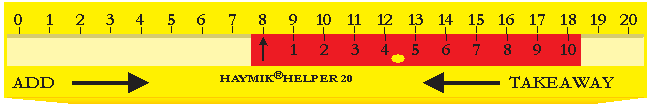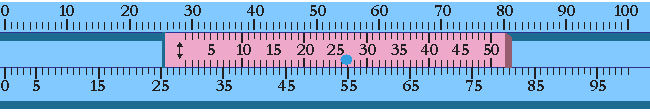Hayden says: “Mikyla I have learnt other strategies such as rounding and compensation for example: to add 28 and 37. 28 + 37 =?
With the arrow on the slide aligned under 28 on the top board, I can see 28 is 2 less than 30 and 37 is 2 more than 35: (28+2) + (37- 2) Rounding 28 up by 2 and compensation by taking 2 from 37
= 65      Sum (answer) to 37+28
We can check our answer to 28+37 on the “Haymik helper” as above:
With arrow on the slide aligned under 28 on the top board, 37 on the slide is aligned over 65 the sum (answer to 28+37) on the bottom Board”.
Mikyla says to Hayden
“I have learnt a different strategy for addition using partitioning (tens)  and Number Properties:for 28 +37 =?
28 +37 = (28 +8) + (30 + 7) Renaming
= 20 + (8 + 30) +7    Associative Property
= 20 + (30 + 8) +7    Commutative Property
= (20 + 30) + (8 + 7) Associative Property